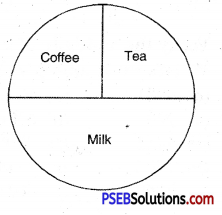# PSEB 4th Class Maths Solutions Chapter 9 Data Handling Ex 9.2

Punjab State Board PSEB 4th Class Maths Book Solutions Chapter 9 Data Handling Ex 9.2 Textbook Exercise Questions and Answers.

## PSEB Solutions for Class 4 Maths Chapter 9 Data Handling Ex 9.2

Question 1.
Given bar graph represents the number of students absent during the week :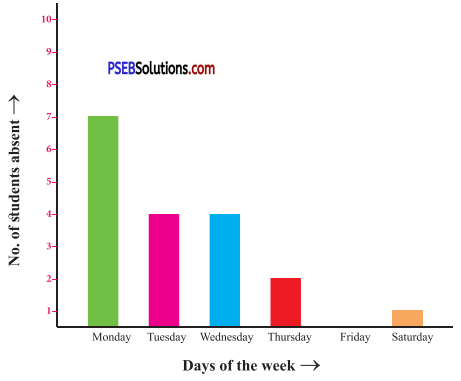(a) Find the number of students class 4 who are absent oh Monday ?
(b) On which day of the week no student was absent ?
(c) On which day of the week the maximum number of students were absent ?
(d) Find the number of students of 4th class who are absent on Friday ?
(e) On which two days of the week the number of absentee students are equal and how many ?
Solution:
(a) 7,
(b) Friday,
(c) Monday,
(d) zero
(e) Tuesday and Wednesday, the number of students absent on these days = 4, 4

Question 2.
Number of students who play different games are given below :
Kho-Kho = 10
Football = 25
Cricket = 20
Draw bargraph representing above data.
(*Scale of 5 children should be taken)
Solution: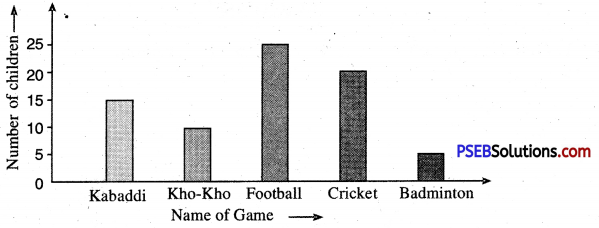Question 3.
There is a T20 cricket match between Australia and India at I.S. Bindra stadium Mohali. India scored following runs in 6 overs of power play.
Over no. 1 = 6
Over no. 2 = 9
Over no. 3 = 3
Over no. 4 = 18
Over no. 5 = 6
Over no. 6 = 12
Solution:
Draw bargarph representing above data.
(Hint: Scale – 3 Runs = 1 unit)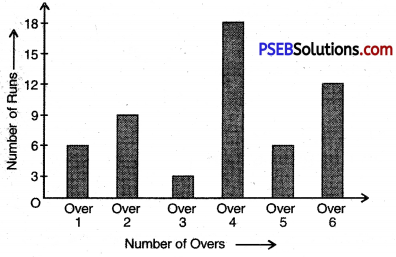Question 4.
Look at the pie chart and answer the following :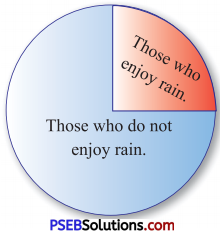(a) How many students come to get drenched in rain ?
(b) How many students do not like to get drenched in rain ?
If there are 32 children in a class.
(c) How many students like to be drenched in rain.
(d) How many students do not like to be drenched in rain.
Solution:
(a) $$\frac{3}{4}$$,
(b) $$\frac{1}{4}$$
(c) $$\frac{3}{4}$$ × 32 = 8
(c) $$\frac{1}{4}$$ × 32 = 8

Question 5.
When the Teacher asked the students of fourth class, what do they like most, Tea, Coffee, or milk, the following table was obtained.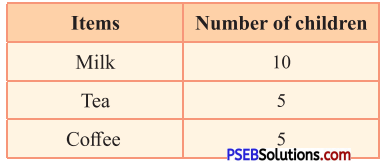Total number of children = ……….
Represent the above data by a pie chart.
Solution:
10 + 5 + 5 = 20.